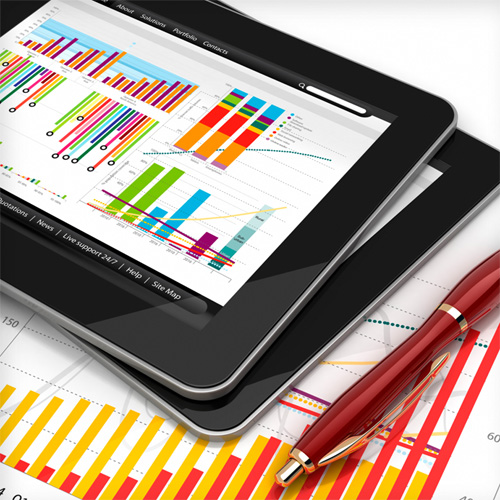Modelling and simulation is a powerful tool for the conceptual design, operational analysis and optimization of energy engineering components, systems and processes. Modelling and simulation activities can reduce product design time and time to market and improve product performance, efficiency, and quality. The aim of this course is to introduce students to the methodologies and tools involved in the modelling and simulation of advanced energy systems in general and PV systems in particular. The students should be able to (1) formulate, mathematically describe, numerically solve and analyse energy conversion processes, using advanced numerical tools and (2) perform techno-economic analyses for the integration of PV units in energy systems.

Learning Outcomes

On successful completion of the course, students will be able to:

• Comprehend the basic principles of modelling and simulation of energy systems.
• Demonstrate the ability to formulate, mathematically describe, numerically solve and analyse solar energy conversion processes, using advanced numerical tools such as CFD.
• Demonstrate the ability to assess the technical and economic performance of PV units and their integration into the energy system.
• Contents:

• Fundamentals of Energy Systems Design (Mass and energy balances, Energy efficiency, Environmental assessment).
• Introduction to Modelling and Simulation (Multiscale physics-based models, Performance-based models, System integration).
• Energy Performance and Financial Feasibility of PV Systems (Energy model, Cost analysis, GHG analysis, Financial analysis)
• Thermal Performance of PV Panels (Problem formulation, Geometry modelling, Meshing, Boundary and initial conditions, Physical models and input parameters, Run simulation, Post-process results)
• Hands-on computations using the ANSYS Student and RETScreen software platforms.